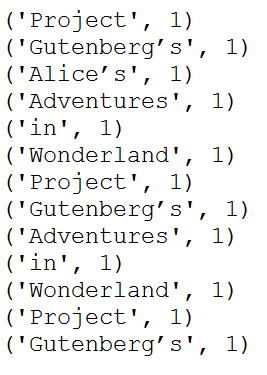# Spark map() Transformation

Spark `map()` is a transformation operation that is used to apply the transformation on every element of RDD, DataFrame, and Dataset and finally returns a new RDD/Dataset respectively. In this article, you will learn the syntax and usage of the map() transformation with an RDD & DataFrame example.

Transformations like adding a column, updating a column e.t.c can be done using map, the output of map transformations would always have the same number of records as input. This is one of the differences between `map()` and flatMap() transformations.

## 1. Spark map() usage on RDD

First, let’s create an RDD from the list.

``````
val data = Seq("Project",
"Gutenberg’s",
"Alice’s",
"in",
"Wonderland",
"Project",
"Gutenberg’s",
"in",
"Wonderland",
"Project",
"Gutenberg’s")

val rdd=spark.sparkContext.parallelize(data)
``````

### 1.1 RDD map() Syntax

``````
map[U](f : scala.Function1[T, U])(implicit evidence\$3 : scala.reflect.ClassTag[U]) : org.apache.spark.rdd.RDD[U]
``````

### 1.2 RDD map() Example

In this `map()` example, we are adding a new element with value 1 for each element, the result of the RDD is PairRDDFunctions which contains key-value pairs, word of type String as Key and 1 of type Int as value.

``````
val rdd2=rdd.map(f=> (f,1))
rdd2.foreach(println)
``````

This yields below output.

## 2. Spark map() usage on DataFrame

Spark provides 2 map transformations signatures on DataFrame one takes scala.function1 as an argument and the other takes Spark `MapFunction`. if you notice below signatures, both these functions returns Dataset[U] but not DataFrame (DataFrame=Dataset[Row]). If you want a DataFrame as output then you need to convert the Dataset to DataFrame using `toDF()` function.

### 2.1 Dataframe map() syntax

``````
1) map[U](func : scala.Function1[T, U])(implicit evidence\$6 : org.apache.spark.sql.Encoder[U])
: org.apache.spark.sql.Dataset[U]
2) map[U](func : org.apache.spark.api.java.function.MapFunction[T, U], encoder : org.apache.spark.sql.Encoder[U])
: org.apache.spark.sql.Dataset[U]
``````

### 2.2 Dataframe map() Example

One key point to remember is these both transformations returns the `Dataset[U]` but not the `DataFrame` (In Spark 2.0,  `DataFrame = Dataset[Row]`) .

``````

val structureData = Seq(
Row("James","","Smith","36636","NewYork",3100),
Row("Michael","Rose","","40288","California",4300),
Row("Robert","","Williams","42114","Florida",1400),
Row("Maria","Anne","Jones","39192","Florida",5500),
Row("Jen","Mary","Brown","34561","NewYork",3000)
)

val structureSchema = new StructType()

val df2 = spark.createDataFrame(
spark.sparkContext.parallelize(structureData),structureSchema)
df2.printSchema()
df2.show(false)

import spark.implicits._
val df3 = df2.map(row=>{
val util = new Util()
val fullName = row.getString(0) +row.getString(1) +row.getString(2)
(fullName, row.getString(3),row.getInt(5))
})
val df3Map =  df3.toDF("fullName","id","salary")

df3Map.printSchema()
df3Map.show(false)
``````

Yields below output after applying map() operation.

``````
root
|-- fullName: string (nullable = true)
|-- id: string (nullable = true)
|-- salary: integer (nullable = false)

+----------------+-----+------+
|fullName        |id   |salary|
+----------------+-----+------+
|James,,Smith    |36636|3100  |
|Michael,Rose,   |40288|4300  |
|Robert,,Williams|42114|1400  |
|Maria,Anne,Jones|39192|5500  |
|Jen,Mary,Brown  |34561|3000  |
+----------------+-----+------+
``````

As you notice the above output, the input of the DataFrame has 5 rows so the result of the map also has 5 but the column counts are different.

### Conclusion

In conclusion, you have learned how to apply a Spark `map` transformation on every element of Spark RDD/DataFrame and learned it returns the same number of elements as input.

### Related Articles

#### Reference

Happy Learning !!

### NNK

SparkByExamples.com is a Big Data and Spark examples community page, all examples are simple and easy to understand and well tested in our development environment Read more ..

### This Post Has One Comment

1.Ganesh

This is really great information.